# Analyze Cylindrical Reflector Antenna with Horn Array Feed

This example shows how to analyze an antenna consisting of a cylindrical reflector with a linear array of horns.

### Create Horn Antenna

Create a horn antenna resonating at 10 GHz. Set `lambda` to the free space wavelength at the design frequency of 10 GHz.

```freq = 10e9; lambda = physconst('light')/freq; h = design(horn,10e9); h.FlareWidth = 0.0416; h.FlareLength = 0.0416; h.FlareHeight = 31.8e-3; h.Length = 21e-3; h.FeedOffset(1) = -3.6e-3; figure; show(h)```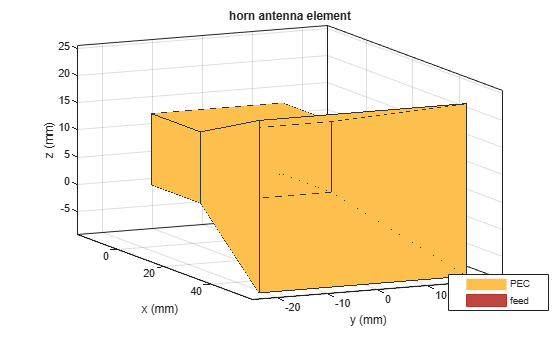### Plot VSWR and Radiation Pattern of Horn Antenna

Plot the voltage standing wave ratio (VSWR) of the horn antenna over the frequency range of 8.5–12.5 GHz.

```range = (0.85:0.01:1.25)*freq; figure; vswr(h,range); ylim([0 5]);```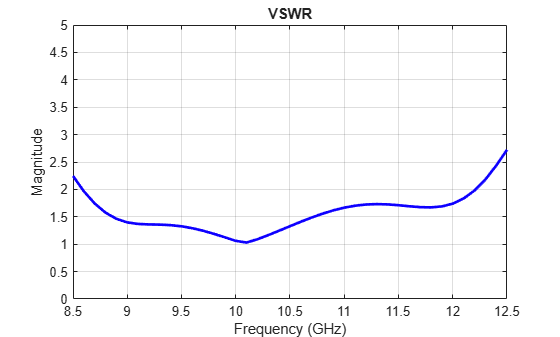The VSWR of the horn antenna is less than 2 between 8.6–12.2 GHz.

Plot the radiation pattern of the horn antenna.

```figure; pattern(h,freq);```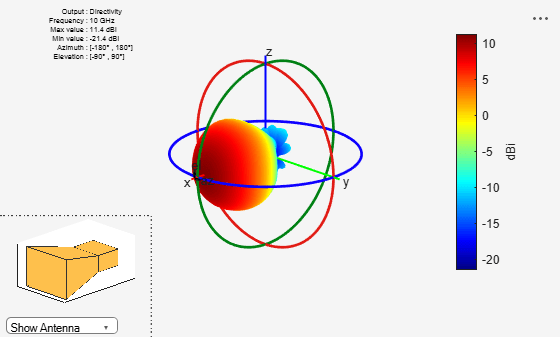The directivity of the antenna is 11.4 dB.

### Animate the Field in the E-plane and H-plane

The analysis from the solver provides us with the steady state result. The field calculation provides the 3 components of the electric and magnetic field in phasor form in the cartesian coordinate system. Animate this field by using a propagator defined by the frequency, fc to bring the result back to time-harmonic form and visualize on the E-plane and H-plane. In the case of the horn, E-plane is the XZ plane and H-plane is the XY plane. As noted in the far-field pattern plot shown in the previous section, the main lobe is along the positive x-axis. Use the helperAnimateEHFields function as shown below. Define the spatial extent, point density as well as the total number of time periods defined in terms of the frequency fc. Choose the Ez component with the plotting plane defined as XZ for the E-field and the Hy component with the plotting plane defined as XY for the animation. If the sampling time is not defined, the function automatically chooses a sampling time based on 10 times the center frequency. There is also an option available to save the animation output to a gif file with an appropriate name specified.

```% Define Spatial Plane Extent SpatialInfo.XSpan = 20*lambda; SpatialInfo.YSpan = 20*lambda; SpatialInfo.ZSpan = 20*lambda; SpatialInfo.NumPointsX = 300; SpatialInfo.NumPointsY = 300; SpatialInfo.NumPointsZ = 300; % Define Time Extent Tperiod = 1/freq; T = 1*Tperiod; TimeInfo.TotalTime = T; TimeInfo.SamplingTime = []; % Define E-Field Data details DataInfo.Component = 'Ez'; DataInfo.PlotPlane = 'XZ'; DataInfo.DataLimits = [-5 10]; DataInfo.ScaleFactor = 1; DataInfo.GifFileName = 'hornEz.gif'; DataInfo.CloseFigure = true; %% Animate helperAnimateEHFields(h,freq,SpatialInfo, TimeInfo, DataInfo) ```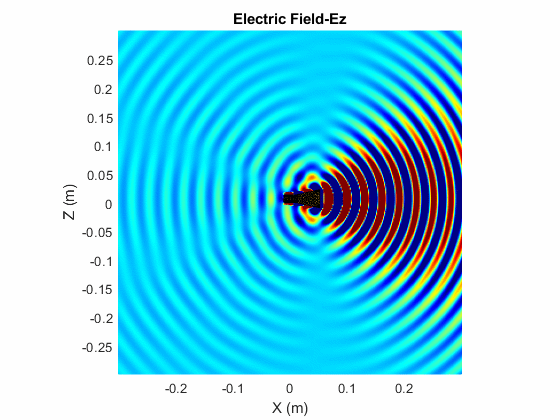```% Define H-Field Data details DataInfo.Component = 'Hy'; DataInfo.PlotPlane = 'XY'; DataInfo.DataLimits = [-0.25 0.5]; DataInfo.ScaleFactor = 1; DataInfo.GifFileName = 'hornHy.gif'; %% Animate helperAnimateEHFields(h,freq,SpatialInfo, TimeInfo, DataInfo) ```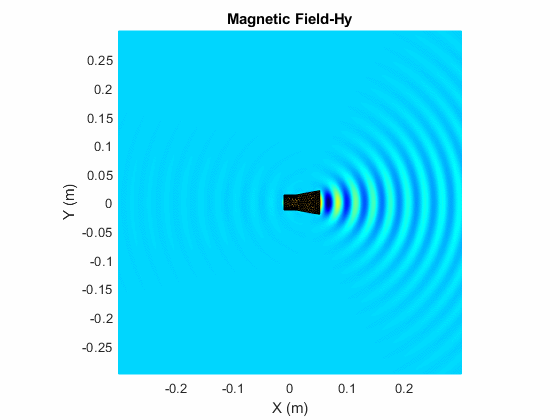### Create Linear Array of Horns

Create a linear array of horns. Set the number of elements in the array to 4 and the spacing between the elements to 1.5 times of lambda.

```arr = linearArray('Element',h,'NumElements',4); arr.Element.Tilt = -90; arr.Element.TiltAxis = 'Z'; arr.ElementSpacing = 1.5*lambda; figure; show(arr)```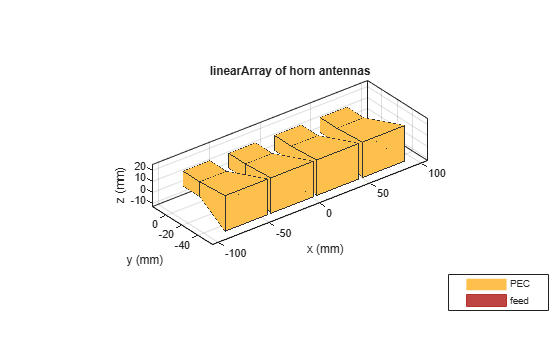### Create Cylindrical Reflector with Horn Array

Create a cylindrical reflector with the linear array of horns as the exciter. Tilt the exciter at an angle of 90 degrees along the Z-axis and 70 degrees along the Y-axis.

```ref = reflectorCylindrical; ref.GroundPlaneLength = 10.2*lambda; ref.GroundPlaneWidth = 10.3*lambda; ref.Spacing = 8.5*lambda; ref.Tilt = 90; ref.Exciter = arr; ref.Exciter.Tilt = [90 70]; ref.Exciter.TiltAxis = ['Z','Y']; figure; show(ref)```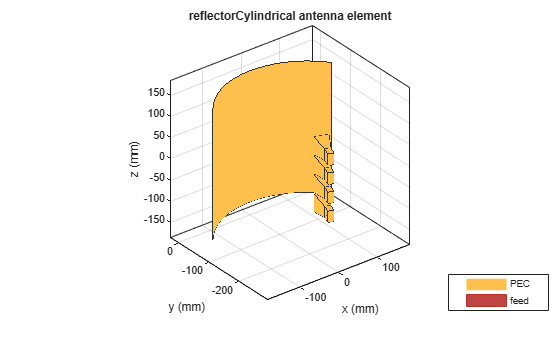### Mesh and Plot Radiation Pattern of Cylindrical Reflector with Horn Array

Mesh the reflector manually with the maximum edge length of `lambda/10`.

```figure; mesh(ref,'MaxEdgeLength',lambda/10);```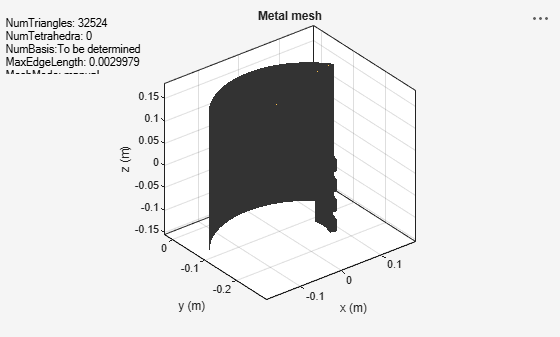Load the solved reflector file `refObj.mat`.

`load("refObj.mat");`

```figure; pattern(ref,freq); view([80 10]);```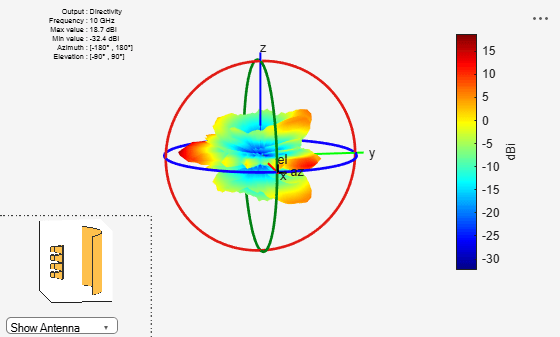You can also plot the radiation pattern without using the MAT file, but the `pattern(ref,freq)` command takes up to 18 minutes to plot the radiation pattern. To run the `pattern(ref,freq)` command, these machine configurations are preferred:

• Processor: Intel ® Xeon ® CPU E5-1650 v4 @3.60GHz.

• RAM: 64GB.

• System type: 64-bit operating system.

The directivity of the cylindrical reflector antenna with a horn array feed is 18.7 dB.